Reach Us+441474556909
Methods of Image Edge Detection: A Review | OMICS International
Journal of Electrical & Electronic Systems
All submissions of the EM system will be redirected to Online Manuscript Submission System. Authors are requested to submit articles directly to Online Manuscript Submission System of respective journal.

# Methods of Image Edge Detection: A Review

Dharampal and Vikram Mutneja*

Shaheed Bhagat Singh State Technical Campus, Ferozepur, Punjab, India

*Corresponding Author:
Vikram Mutneja
Shaheed Bhagat Singh State Technical Campus
Ferozepur, Punjab, India
E-mail: [email protected]

Received Date: March 22, 2015; Accepted Date: July 07, 2015; Published Date: July 30, 2015

Citation: Dharampal, Mutneja V (2015) Methods of Image Edge Detection: A Review. J Electr Electron Syst 4:150. doi:10.4172/2332-0796.1000150

Copyright: © 2015 Dharampal, et al. This is an open-access article distributed under the terms of the Creative Commons Attribution License, which permits unrestricted use, distribution, and reproduction in any medium, provided the original author and source are credited.

Visit for more related articles at Journal of Electrical & Electronic Systems

#### Abstract

The first step in an image recognition system is the edges sensibility in a digital image. Edge detection for object observation in image processing is the important part. This will give us a good understanding of edge detection algorithms. An edge is useful because it marks the boundaries and divides of plane, object or appearance from other places things. For pattern recognition it is also an intermediate step in the digital images. An edge consists of pixels with the intensity variations of gray tones which are different from their neighbour pixels. This paper introduces the standard edge detection methods which are widely used in image processing such as Prewitt, Laplacian of Gaussian, Canny, Sobel, Robert and also the new approach are discussed in this known as Fuzzy logic.

#### Keywords

Edge detection; Fuzzy logic; Gradient magnitude; Laplacian operator; Robert; Sobel; Prewitt; Canny

#### Introduction

An edge may be the result of changes in light, colour, shade and texture, and these changes can be used to resolve the depth, size orientation and surface properties of a digital image. Digital analysis of the image helps to filtering unnecessary information to select the edge points. The detection of refined changes may be mixed up by noise and this depends on the pixel threshold of change that defines an edge. Detection of these edges is very difficult and time consuming especially when an image is corrupted by noise. Edge detection is a basic and important tool in the main areas of image processing such as feature detection and feature extraction .

Shashank Mathur and Anil Ahlawat , proposed a edge detection algorithm using windowing technique. This is based on fuzzy relative pixel values in the 3*3 pixels mask for scanning of image. Their technique based to a set of fuzzy condition used to check the pixel magnitude gradient in the window by made comparison of pixel values with adjacent pixels.

Yasar Beceriklil and Tayfun , proposed fuzzy rule based algorithm provide the flexibility in handling edge thickness in the processed image. They studied that edge detection which is use full is used in image segmentation, registration and identification purposes. Variation of intensity/gray level defines their shape and size. Various edge profiles are shown in Figure 1.

Standard methods of edge detection are involves convolving the image with an operator. This made an operator to be sensitive to large gradients in the image while returning values of zero in uniform regions [4,5].

#### Edge Detection

Edge detection can be defined as the discovery of lines that marks the limit and divides of image appearance from other places or things in a digital image .

Edge detection uses an approach where the intensity variations occur in the image points is declared as the edge. It is a series of actions used to identify the points in an image where clear and defined changes occur in the intensity. This series of action is necessary to extract the image related information e.g. image sharpening, enhancement and object location present in the image.

Flow chart for edge detection

Edge detection algorithm

1. Firstly, take a colour image.

2. Refining: Refining is used to remove the noise as possible without the damage of the true edges of it.

3. Intensification: Apply differentiation to enhance the quality of edges.

4. Threshold: Edge magnitude threshold is used to reject the noisy edge pixels and other should be confined.

5. Localization: Some applications to estimate the location of an edge and spacing between pixels, sub pixels resolution might be required.

6. Get the image after edge exposures.

Approaches of edge detection

The method for edge detection is classified into two categories; first is gradient based and second is Laplacian based.

In the gradient based method edges are detected by taking the first order derivative of the image. The gradient magnitude is used to calculate a measure of edge strength. Gradient magnitude has the properties of local directional maxima helps in computed local edge orientation which is in the gradient direction [7,8].

In the laplacian based method, image is used to compute the second order derivative expression which has a zero crossing. Generally, edges are found by searching zero crossing of a non linear differential expression. Typically for edge detection a pre processing step Gaussian smoothing is applied which is commonly a refining stage [7,8].

Gradient based edge detection

The gradient based approach is also called as mask in digital images. Differential approximations in the either horizontal or vertical direction of the image are calculated with the digital mask .

The edge part of the image declared where the gray value rapidly changes. The first order derivative of the image is used to find out the maximum and minimum values in the gradient based operator [4,7]. In image processing using the magnitude of the gradient first order derivatives is achieved . For a function f (x, y), as the dimensional column vector the differential of ‘f’ at coordinates (x, y) is denoted  as given below:(1)

Where ∇f is the gradient of vector. The (x, y) coordinates have the maximum rate of change, normally the gradient direction . This can be observed with the vector evaluation. Here the angle is represents with the gradient angle (Figure 2).

Magnitude of vector ∇f, denoted as M(x, y):

M (x, y) = magnitude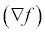=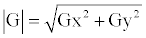(2)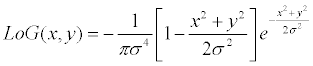The square root operation is used to approximate this quantity.

M (x, y) = Gx2 + Gy2 (3)

Or the complete values using,

M (x, y) ≈ Gx + Gy (4)

The gradient direction is given as: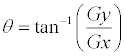(5)

Here the angle is measured with citation to x- axis. The direction of edge is perpendicular to the gradient at that point. The gradient of the 2D image  is given as:

Gx = f (x +1, y) − f (x, y) (6)

And

Gy = f (x, y +1) − f (x, y) (7)

In gradient based method high gradient pixels are accepted as edges. An intensity derivative at some direction considered at edge pixels given by the angel of the gradient vector. Let Figure 3, which denotes the intensities of image points in a 3*3 region.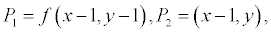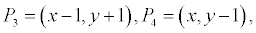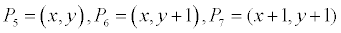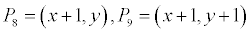In this process, we will learn gradient based operator such as, Sobel, prewitt, and Laplacian of Gaussian operator

(1) Robert operator: This operator consists of 2*2 mask of simple contiguity.

These two masks can be applied separately to the image. This analysis can obtain the results in the directions of Gx and Gy. Perpendicular and parallel direction  is determined respectively: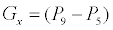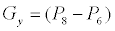(8)

The even size masks are difficult to implement because they do not have a centre of uniformity . Further above equation can be written as follows:(9)

The gradient of magnitude is given as: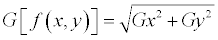(10)

The approximation magnitude is given as: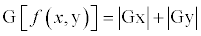(11)

Here Gx and Gy are calculated using the masks shown in Figure 4. The edge with the angle of orientation giving rise to the special gradient is given as:

θ = arctan arctan(Gy / Gx) − 3 / 4 (12)

Sobel Operator: In edge detection methods Sobel operator is widely used .

This operator can be implemented by filtering an image with the kernel or left mask. To perform the square of pixels values image is again filtered with other mask. Now the two results are add their root is computed. The 3*3 convolution masks for sobel are shown in Figure 5.

We can compute the approximation of the partial derivative in x-direction by taking the difference between the 3rd and 1st rows of 3*3 image region is implemented with the use of left mask or kernel of Figure 5. The other mask in y-direction is implemented by taking the difference between the 3rd and 1st columns to approximate the derivative .

Here the partial derivatives are given as: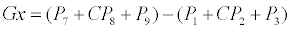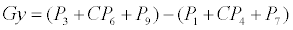(13)

With the value hold constant c=2. Further the equation can be given as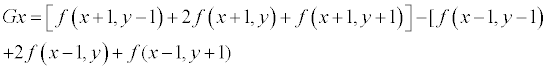(14)

And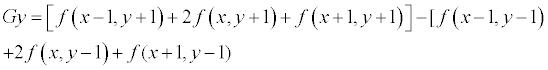(15)

The gradient of magnitude is given as:(16)

The edge has an angle of orientation, which boost up the spatial gradient is given as: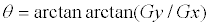(17)

Prewitt Operator: As the Sobel operator uses the same equations are used by it with the constant c=1. So that mask for the Prewitt operator shown in Figure 6. It is similar to Sobel operator . In Sobel operator, pixels that are closer to the centre of the masks do not place any emphasis on it . Traditional edge detection methods are used mostly because they are simple and detection of edges and their orientation is easily possible. These operators [15,16] are sensitive to noise, and are imprecise [17-23].

Canny Operator: The other name of this algorithm is an optimal edge detector  by using this algorithm the edges of the image is found with applying the aptness without emotive the features of the edges, so that canny is a very important technique to find edges .

The algorithm for canny edge operator is given as follows:

1. We can get the smooth image k^(s, t), by convolve the image k (s, t) with a Gaussian function.

k ^ (s,t) = k (s,t )*G(s,t,6)

2. To compute edge strength apply first difference gradient operator, then edge magnitude and direction are obtained.

3. Apply gradient magnitude with the non-maximal and suppression.

4. Then on the output suppression image apply the threshold of the non-maximal .

#### Edge Detection Method Based on Laplacian

The Laplacian based edge detector found image edges by compute the second order derivative expression of the image. The second order expression has zero crossing where the image edges are found .

However, Laplacian of Gaussian is very useful because the 2nd derivative is very sentient of noise and this is helpful in the filtering of noise from the image .

Laplacian of gaussian (Log)

The Laplacian of Gaussian edge detector is also referred as LoG. It is also known as Marr-Hildreth edge detector .

This approach defines the image is convoluting with Gaussian filter is to reduce the noise after that smoothing is used to filter out the isolated noise points and the related small structures (Figure 7). Those pixels, that have locally maximum gradient, are examined as edges in which the zero crossing is found [14,26,27]. The zero crossing of second order derivative expression avoid the unimportant edges whose correlate with first order derivative selected image edges of those pixel have value above some threshold. Edge direction can obtain where the zero crossing occurs . The 2D LOG function [29,30] which is cantered on zero-crossing and the σ standard Gaussian deviation has given in the form: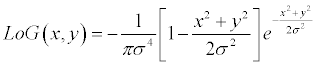Here, we show the LoG function with the three dimensional plot . Its view looks like the Mexican hat as shown in Figure 8.

The Laplacian edge detector uses only one mask to compute the second order derivative mask unlike the Sobel operator. The mask used for it is shown in Figure 9.

During the run time on the image from the above three used only one mask because the mask in LoG operator can be calculated in advance . We can find the correct location of edges in all directions by testing the area around the pixels for those have fixed attributes [17-23]. The orientation of edges and their detection are possible in LoG operator. The variations in the gray level pixels intensity are the indication of the correct edge places, but sometimes there is the damage at the corners and curves. It does not respond to some existing edges because it is also a sensation of noise [31-33].

Edge detection based on fuzzy logic

The decision making is to be full filled by the use of fuzzy logic based approach. Since the concept of fuzzy logic was developed in 1965 by Zadesh. In the image processing areas, many analysis have been carried out some of them image edge detection, segmentation and quality assessment. In the past, researchers suggested many techniques for edge detection with the use of fuzzy logic .

Davesh Nawgaje  presented a Fuzzy Inference System (FIS) approach to detect the edges of the microscopic images within colour, which is robust and has stability degrees. They proposed the logic based technique which is a set of three pixels and also used the smallest mask of 2*2 window image consists of a set of fuzzy rules which highlight all the edges that are correlated with an image (Figure 10).

Image processing based on fuzzy logic: Fuzzy logic based image processing is helpful in many fields of processing an image such as feature extraction, segmentation, enhancement and edge detection. This is classified into following three stages:

1. Fuzzification of image

2. Membership values alteration

3. Deffuzification of image

For the image fuzzification and deffuzification there is no need of the hardware because the coding and decoding of the image data is possible during the processing of images.

In the image processing fuzzy logic helps in the various fields of applications. It supports the if-then rules which are based on human knowledge in the form of coding and to overcome the difficulties use of expert knowledge is possible. It consists of fuzzy set theory which is a powerful tool.

There are the different approaches for edge detection is used, some of them are:

Yasar Beceriklil and Tayfun Karan  proposed that we can apply the heuristic rules to the system and results were checked for different images. Moreover system is consists of dynamic structure adopted by the variation in rules easily. Dharampal et al. , proposed an improved type-1 fuzzy logic method for edge detection. This paper applies the Sobel operator combined with a type-1 fuzzy inference system (T1FIS) where the authors conclude that T1FIS is better than the standard methods of edge detection.

In the classical techniques for edge detection with the certain parameters such as threshold and σ is used and have fixed edge thickness. But the fuzzy rules base offers the more advantages such as by adding the new rules or by changing parameters we can change the edge thickness so that’s why it is a flexible structure and can be adopted any time to implement edge detection process.

The fuzzy based edge detection method provides the flexible structure and less complexity, so that in future, we will detect the edges using the fuzzy rule base.

#### References

Select your language of interest to view the total content in your interested language

### Article Usage

• Total views: 30669
• [From(publication date):
September-2015 - Aug 25, 2019]
• Breakdown by view type
• HTML page views : 25525
• PDF downloads : 5144

## Post your commentCan't read the image? click here to refresh
###### Peer Reviewed Journals

Make the best use of Scientific Research and information from our 700 + peer reviewed, Open Access Journals

International Conferences 2019-20

Meet Inspiring Speakers and Experts at our 3000+ Global Annual Meetings

Top